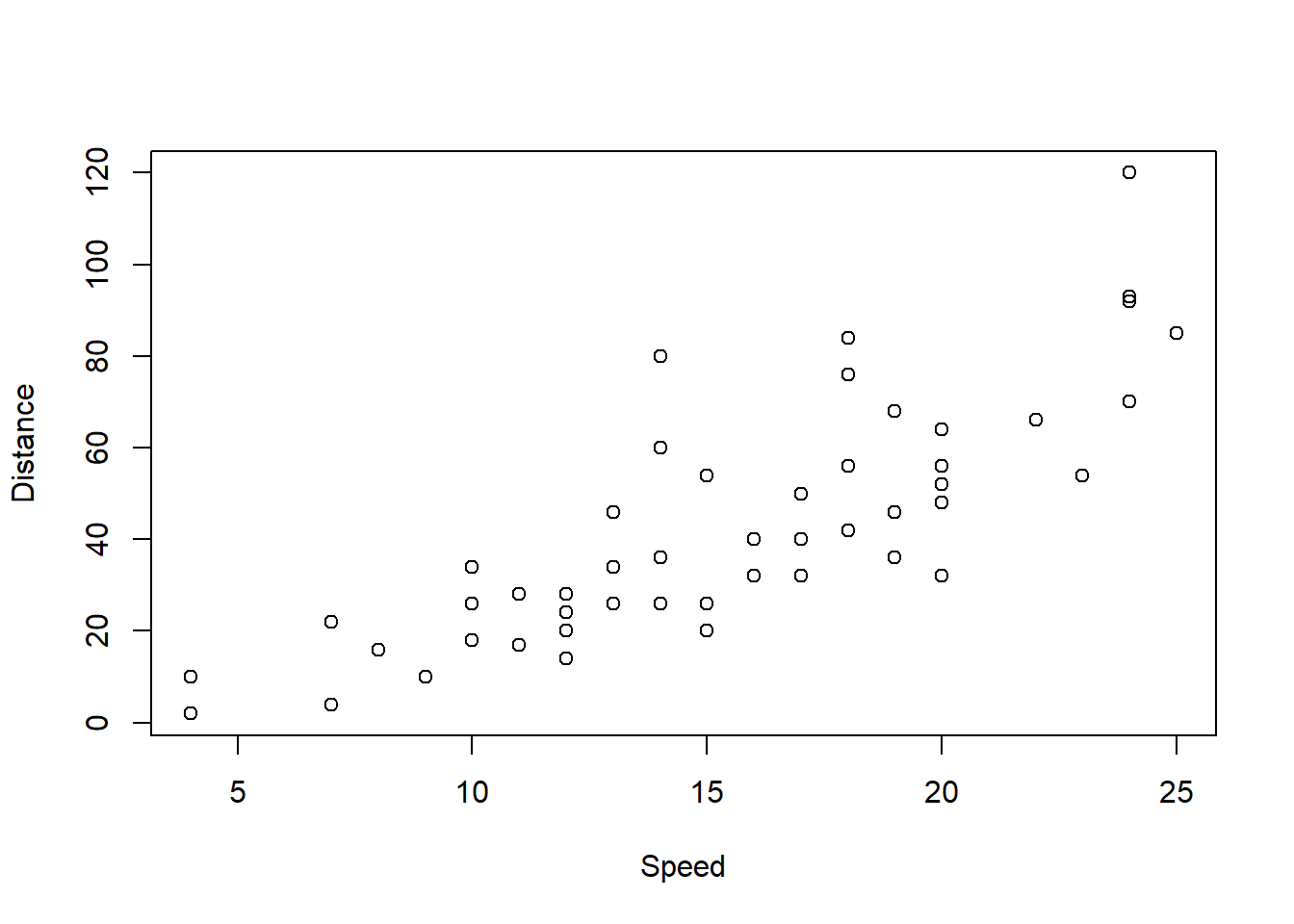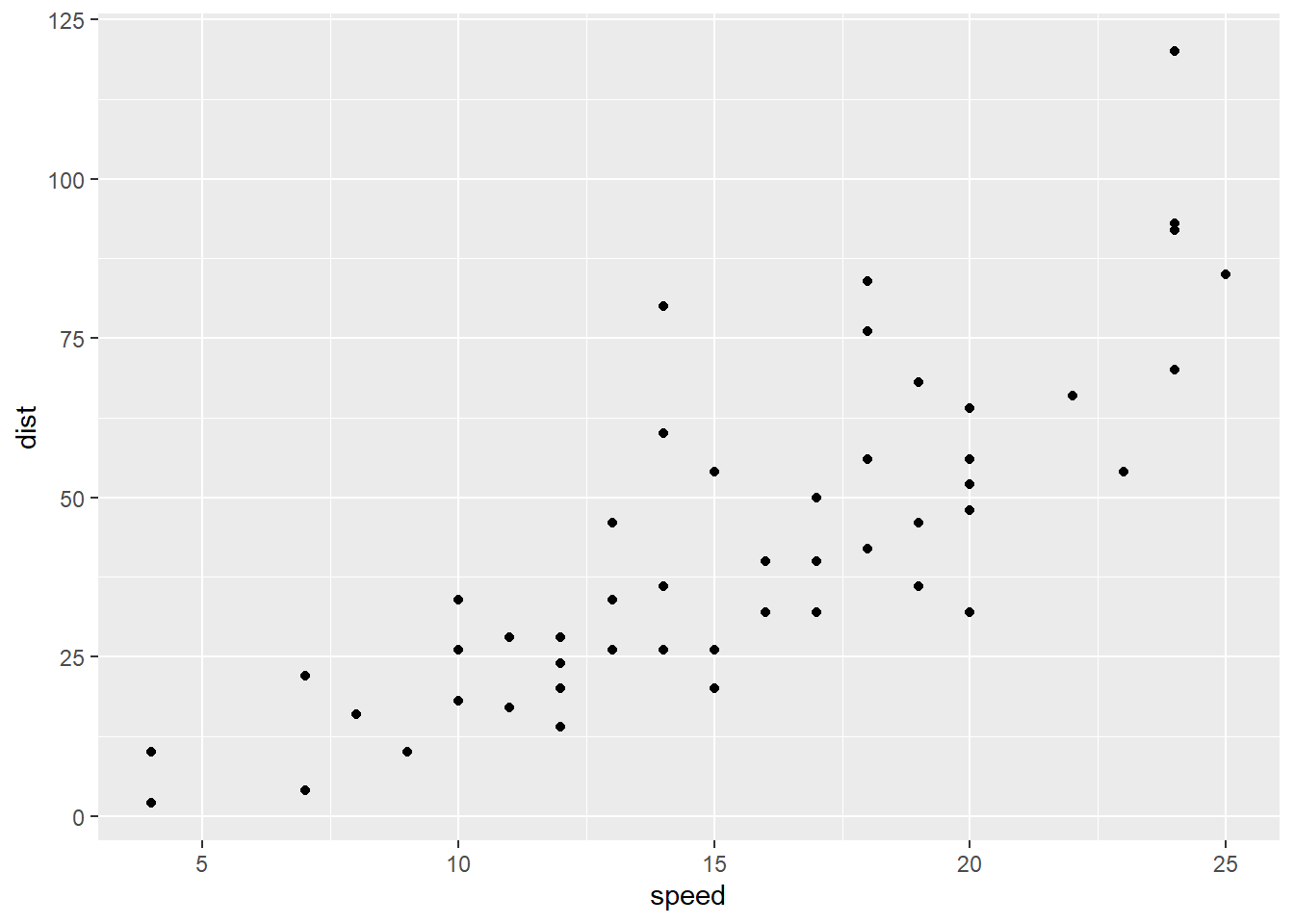### Learning objectives

• Install R
• Learn how to run basic commands

### Content

• Learn about R and Rstudio
• Install R and Rstudio
• RStudio interface
• Simple commands in the console
• Write and read excel/text files
• Select row/column in dataframe
• Summary statistics
• Simple plot
• Install and call libraries
• First look at ggplot

### What is R?

R is like any other programming language that is used for statistical computing and graphics. R is an open source tool by R Foundation for Statistical Computing. Learn more about the background of R at https://www.r-project.org/about.html.

### What is RStudio?

RStudio is an integrated development environment (IDE) for R. Where IDE is a software application that provides facilities to run codes, develop softwares and many other things. We will use Rstudio to write/run codes, visualize graphs and do other analysis. Learn more about Rstudio at https://rstudio.com/about/

### RStudio interface

RStudio IDE consists of numerous options. I find the following cheat sheet nicely summarizes the IDE options available https://rstudio.com/wp-content/uploads/2016/01/rstudio-IDE-cheatsheet.pdf

### Simple commands in the console

RStudio console can be used to write/run codes. Couple of examples are shown below. Run each of the examples in the console. You can see the variables “a” and “b” are stored in the environment panel that is generally located on the right side of RStudio IDE.

#### Example 1

Store single values in variable a and b, then do simple calculations.

a = 5
b = 2
a+b
##  7
(a+b)^2
##  49

#### Example 2

Create series and store in variable. This is also known as vector.

a = 1:10 # 1 to 10 at interval 1
print(a)
##    1  2  3  4  5  6  7  8  9 10
b = seq(1,22,3) # 1 to 22 at interval 3
print(b)
##   1  4  7 10 13 16 19 22
print(b^2)
##    1  16  49 100 169 256 361 484

#### Example 3

Create 2D data that has rows and columns. It can be dataframe or matrix. Lets now just work with dataframe. Dataframe is a 2D array-like structure that can store numeric, factor or character type. More about dataframe can be found here https://www.tutorialspoint.com/r/r_data_frames.htm

a = 1:10
b = 11:20
df = data.frame(col1 = a, col2 = b) # storing a and b as two columns
head(df, 4) # head() is used to see first few lines (here 4) of dataframe
##   col1 col2
## 1    1   11
## 2    2   12
## 3    3   13
## 4    4   14

### Write and read excel/text files

Here we will used an already existing dataset in R to write/read examples. The dataset is stored in the variable “cars”, which has two columns: speed and distance. Below, two examples shown where the example 1 shows how to write data in excel/txt format, and example 2 shows how to read those dataset. Before the examples, the following codes can be used to change the working directory, where working directory is the default folder in your computer where exported/imported files are located.

#setwd() command changes the working directory. Always use /

#check current directory location using getwd()
getwd()
##  "C:/sarfaraz/Project_R_advance/R-tutorial/R_beginner_part1_files"
#to list all the files in the directory
list.files()
##   "cars_example.csv"       "cars_example.txt"       "figure-markdown_github"
##   "figure_test1.png"       "figure_test2.png"       "R_beginner_part1.html"
##   "R_beginner_part1.md"    "R_beginner_part1.R"     "R_beginner_part1.Rmd"
##  "R_beginner_part1_files" "test.png"

#### Example 1

head(cars) # check first few lines of cars variable. Here, cars is a default dataset in R.
##   speed dist
## 1     4    2
## 2     4   10
## 3     7    4
## 4     7   22
## 5     8   16
## 6     9   10
#check the class of cars
class(cars)
##  "data.frame"
#write into an excel file
write.csv(cars, file = 'cars_example.csv')

#write into text file where the values are separated by comma
write.table(cars, 'cars_example.txt',sep = ",")

#### Example 2

#read an excel file

#read text file. where header = T means the first row is the title of each column. sep specifies what is used in the text to separate columns.
head(df2)
##   speed dist
## 1     4    2
## 2     4   10
## 3     7    4
## 4     7   22
## 5     8   16
## 6     9   10

### Select row/column in dataframe

#show row 4 of cars.
a = cars[4,]
print(a)
##   speed dist
## 4     7   22
#show column 1 of cars
b = cars[,1]
print(b)
##    4  4  7  7  8  9 10 10 10 11 11 12 12 12 12 13 13 13 13 14 14 14 14 15 15
##  15 16 16 17 17 17 18 18 18 18 19 19 19 20 20 20 20 20 22 23 24 24 24 24 25
#can also select by column name
a2 = cars\$speed
print(a2)
##    4  4  7  7  8  9 10 10 10 11 11 12 12 12 12 13 13 13 13 14 14 14 14 15 15
##  15 16 16 17 17 17 18 18 18 18 19 19 19 20 20 20 20 20 22 23 24 24 24 24 25
#show the value with row = 5, colum = 2
c = cars[5,2]
print(c)
##  16
#show all with speed > 4
d = cars[cars[,1]>4,]
head(d,5) # show only first 5 rows, there are many more
##   speed dist
## 3     7    4
## 4     7   22
## 5     8   16
## 6     9   10
## 7    10   18
#show all with speed > 4 and <9
e = cars[cars[,1]>4 & cars[,1]<9,]
print(e)
##   speed dist
## 3     7    4
## 4     7   22
## 5     8   16
#show all with speed > 4 and dist<16
f = cars[cars[,1]>4 & cars[,2]<16,]
print(f)
##    speed dist
## 3      7    4
## 6      9   10
## 12    12   14
#check the dimension
dim(f) # the first value is row and the second value is column number
##  3 2

### Summary statistics

Summary of the data can be found using summary() command.

#summarize cars
summary(cars)
##      speed           dist
##  Min.   : 4.0   Min.   :  2.00
##  1st Qu.:12.0   1st Qu.: 26.00
##  Median :15.0   Median : 36.00
##  Mean   :15.4   Mean   : 42.98
##  3rd Qu.:19.0   3rd Qu.: 56.00
##  Max.   :25.0   Max.   :120.00
#it is also possible to get specific stat. E.g.
mean(cars[,1]) # mean of all values in the first column
##  15.4
median(cars[,2]) # median of all values in second column
##  36

### Simple plot

Simple scatterplot can be obtained using plot() function. For example,

x = cars[,1]
y = cars[,2]
plot(x,y, xlab=c('Speed'), ylab = c('Distance'))### Install and call libraries

A lot of the functions used in R is not present by default. We have to install packages and call the required libraries to actually use it. For example, the following code shows how to install and call ggplot2 package.

# install.packages('ggplot2') # uncomment the line to execute it. I am commenting it out as it is already installed in my computer.
# install.packages('dplyr')

#call library
library(ggplot2)
library(dplyr)

### First look at ggplot

ggplot is an incredibly powerful tool for plotting. It deserves a separate session to go over it. For now, let us plot the cars dataset using ggplot.

p = cars %>%
ggplot(aes(x = speed, y = dist))+
geom_point()

print(p)Export plot in png format. Image can simply be exported using png() function

png('figure_test1.png')
print(p)
dev.off()
## png
##   2

Plots prepared using ggplot can also be exported using ggsave() function

ggsave(p,filename='figure_test2.png',
width = 20, height = 15, units = "cm")